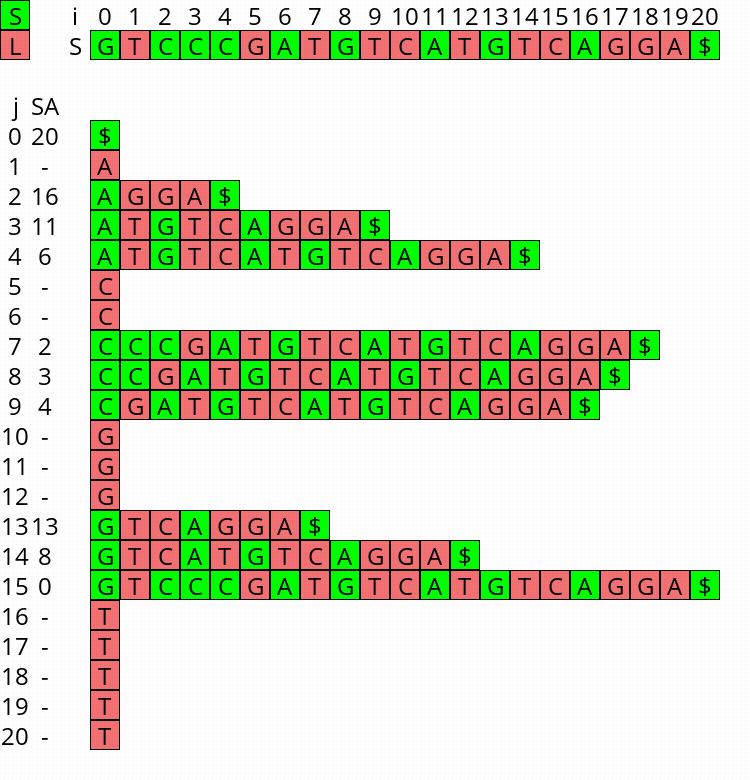# Linear-time suffix array construction

These are some notes about linear time suffix array (SA) construction algorithms (SACA’s).

History of suffix array construction algorithms:

• 1990 first algorithm: Manber and Myers (1993)
• 2002 small/large suffixes, explained below: Ko and Aluru (2005)
• 2009 recursion only on LMS suffixes: Nong, Zhang, and Chan (2009)

These slides from Stanford are a nice reference for the last algorithm.

## Notation

• String $$S = s_0\dots s_{n-1}s_n$$.
• \$s_n=\$$is the sentinel, smaller than all other characters. • Suffix from $$i$$: $$S_i := s_i\dots s_n$$. • $$i$$ indexes the string $$S$$. • $$j$$ indexes the suffix array $$A$$. • Let $$P_i$$ be the position of suffix $$S_i$$ in the suffix array. • $$A_{P_i} = i$$ and $$P_{A_j}=j$$ by definition. • The previous-suffix and next-suffix of $$S_i$$ are $$S_{i-1}$$ and $$S_{i+1}$$ respectively. ## Small and Large suffixes • Suffix $$S_i$$ is small (S-suffix) when $$S_i < S_{i+1}$$. $$S_n$$ is small by definition. • Suffix $$S_i$$ is large (L-suffix) when $$S_i > S_{i+1}$$. • Equality isn’t possible because of the sentinel. Then by definition: • when $$S_i$$ is small: $$P_i < P_{i+1}$$, ie S-suffixes come before their next-suffix. • when $$S_i$$ is large: $$P_i > P_{i+1}$$, ie L-suffixes come after their next-suffix. Lemma 1: When an S-suffix and L-suffix start with the same character $$c$$, the L-suffix comes before the S-suffix in the suffix array. Proof: • The S-suffix is of the form c^x d …, with $$d>c$$ and $$x>0$$. • The L-suffix is of the form c^y b …, with $$b<c$$ and $$y>0$$. • Since $$b<c<d$$, no matter what $$x$$ and $$y$$ are, the L-suffix is less than the S-suffix. ## Building the suffix array from a smaller one It turns out we can build the SA of $$S$$ once we know the SA-order of all small suffixes. Lemma 2: The set of L-suffixes \{S_i : s_i =c \text{ and S_i is large }\} starting with the same character $$c$$ occurs in the same order in the SA as the corresponding next-suffixes $$S_{i+1}$$. Proof: This follows simply from the fact that a set of strings starting with $$c$$ can be sorted by only comparing them from the second character onward. Extension algorithm: This gives the following algorithm to build $$A$$ from the partial suffix array of small suffixes: 1. Start by initializing a bucket for each character $$c$$ in $$S$$: Determine the position in $$A$$ of the first and last suffix starting with $$c$$ by counting the number of characters in $$S$$ that are $$<c$$ and $$\leq c$$ respectively. 2. By Lemma 1, the S-suffixes starting with character $$c$$ come after the L-suffixes starting with $$c$$, and hence can be copied in their already-sorted order to the end of bucket $$c$$. 3. Now we iterate over the suffix array position $$j$$ from $$0$$ to $$n$$ to fill in the large suffixes in the right places. Recall that $$S_{A_j}$$ is the suffix at position $$j$$ of the suffix array, and $$S_{A_j-1}$$ is its previous-suffix. Also, $$A_0 = n$$ by definition, since S_n = \$$ is minimal.
4. If the previous-suffix $$S_{A_j-1}$$ is small, it is smaller than $$S_{A_j}$$ and hence already positioned at a smaller position $$j’ = P_{A_j-1} < j$$.
5. If the previous-suffix $$S_{A_j-1}$$ is large, this is the next-smallest suffix starting with character $$c=s_{A_j-1}$$. Indeed, by Lemma 2, any smaller L-suffix starting with $$c$$ has a smaller next-suffix, which has been processed already (seen at position $$< j$$), while any larger L-suffix has a larger next-suffix, which has not been processed already (not seen at position $$\leq j$$).
Write $$A_j-1$$ to the first empty slot in the bucket for $$c$$.

Once $$j$$ reaches $$n$$, this algorithm has completely filled in $$A$$. Note that it can never run into empty slots.1

## Visualization

I wrote a small visualizer for the algorithm above, see this repo and this post. You can use it to visualize the algorithm for any input string.Figure 1: Constructing the complete suffix array starting after step 2 above, with the small suffixes already filled at the ends of their buckets.
Ko, Pang, and Srinivas Aluru. 2005. “Space Efficient Linear Time Construction of Suffix Arrays.” Journal of Discrete Algorithms 3 (2–4): 143–56. https://doi.org/10.1016/j.jda.2004.08.002.
Manber, Udi, and Gene Myers. 1993. “Suffix Arrays: A New Method for on-Line String Searches.” Siam Journal on Computing 22 (5): 935–48. https://doi.org/10.1137/0222058.
Nong, Ge, Sen Zhang, and Wai Hong Chan. 2009. “Linear Suffix Array Construction by Almost Pure Induced-Sorting.” 2009 Data Compression Conference, March. https://doi.org/10.1109/dcc.2009.42.

1. Proof omitted. ↩︎